# Edward G. Dunne

## Email: egd@ams.org

It is an old (and well-understood) problem in music that you can't tune a piano perfectly. To understand why takes a tiny bit of mathematics and a smattering of physics (acoustics, namely).

There is a PDF version of this article available, which is slightly modified from the original text.

You can also read a more polished version in the article:
Edward Dunne and Mark McConnell, Pianos and Continued Fractions, Mathematics Magazine, vol. 72, no. 2 (1999), 104-115.

### The physics

Let me begin by explaining the way a scale is constructed. To avoid sharps and flats (and to make the diagrams easier to draw), I'll use the key of C. So-called middle C represents a particular frequency. There are various standards for fixing the starting frequency. (I will let the musical directors of orchestras on the two sides of the Atlantic hash out the question of what is actually appropriate...) Also, I will play the old trick of defining my units so that my middle C has a frequency of 1.

There are two pieces of acoustics that matter now:

1. Going up one octave doubles the frequency. Thus, the C one octave up from middle C has a frequency of 2.
2. Tripling the frequency moves to the perfect fifth in the next octave. In our case, this means that the G in the next octave has a frequency of 3.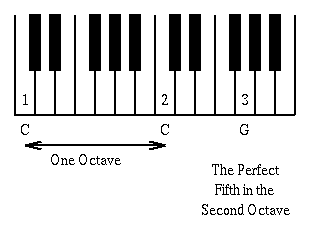By inverting the rule that says that the note one octave than another must have double the frequency, we can fill-in the perfect fifth in the first octave. It should have half the frequency of the G in the second octave.Following Pythagoras, we can now attempt to use these two rules to construct `all the notes', i.e., a complete chromatic scale.

The perfect fifth in the key of G is D. Thus we have, by tripling then halving, then halving again: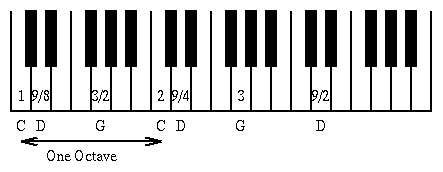Repeat again: the perfect fifth in the key of D is A: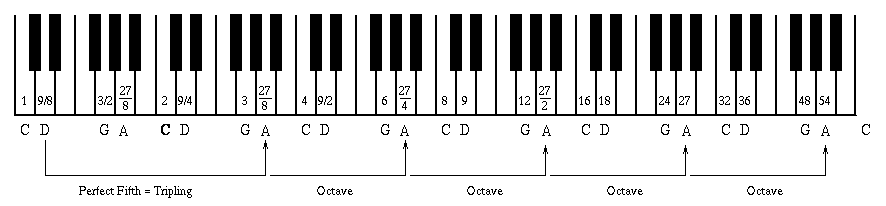We can shorten this by looking at the Table of Fifths, also known as the Circle of Fifths:

Tonic Fifth Tonic
C D E F G A B C
G A B C D E F# G
D E F# G A B C# D
A B C# D E F# G# A
E F# G# A B C# D# E
B C# D# E F# G# A# = Bb B
F# G# A# = Bb B C# D# E# = F F#
C# D# E# = F F# G# A# = Bb B# = C C#
G# A# = Bb B# = C C# D# E# = F G G#
D# E# = F G G# A# = Bb B# = C D D#
A# = Bb B# = C D D# E# = F G S A#
E# = F G A A# B# = C D E E# = F
C D E F G A B C

If we use the rule of doubling/halving for octaves, we arrive at the following frequencies for the twelve notes in our basic octave:

FrequencyTonic Fifth Tonic
1 C D E F G A B C
3 / 2 G A B C D E F# G
9 / 8 D E F# G A B C# D
27 / 16 A B C# D E F# G# A
81 / 64 E F# G# A B C# D# E
243 / 128 B C# D# E F# G# A# B
729 / 512 F# G# A# B C# D# E# = F F#
2187 / 1024 C# D# E# = F F# G# A# B# = C C#
6561 / 4096 G# A# B# = C C# D# E# = F G G#
19683 / 8192 D# E# = F G G# A# B# = C D D#
59049 / 32768 A# B# = C D D# E# = F G S A#
177147 / 131042 E# = F G A A# B# = C D E E# = F
531441 / 262144 C D E F G A B C

However, there are some rules of acoustics that might also be used:

• The frequency of the perfect fifth is 3/2 that of the tonic.
• The frequency of the tonic at the end of the octave is twice that of the original tonic.

• The frequency of the perfect fourth is 4/3 that of the tonic.
• The frequency of the major third is 5/4 that of the tonic.
• The frequency of the minor third is 6/5 that of the tonic.

Using these rules and combining them as efficiently as possible, one arrives at the following list of frequencies for the notes in the C major scale. (That is, I'm leaving out five of the notes from the chromatic scale.)

Note Acoustics Up by fifths, down by octaves
C 1 1
D 9/8 9/8
E 5/4 81 / 64
F 4/3 177147 / 131042
G 3/2 3/2
A 5/3 27 / 16
B 15/8 243 / 128
C 2 531441 / 262144

Notice that some of these fractions are not equal! In particular, the final C in the scale ought to have frequency twice the basic C. Instead, if we go waaaay up by fifths, then back down again by octaves, we have this strange fraction, whose decimal approximation is: 2.027286530. If we took half of this to return to our starting point, we'd have:

531441/524288 = 1.013643265.
This discrepancy is known as the Pythagorean (or ditonic) Comma.

So what is the problem? To answer this, it is time to consider some mathematics.

### The mathematics

The essence of the comparison is that we went up twelve perfect fifths, which is equivalent to changing the starting frequency from 1 to. This should produce another copy of the note C, but seven octaves up. Thus, we should compare this frequency with.

The problem is that we are mixing a function based on tripling (for the fifths) with a function based on doubling (for octaves). More abstractly, we are trying to solve an equation of the type: 2^x = 3^y, where x and y are rational numbers. (With minor finagling, we could restrict to just integers.)

Notice that for different notes in our chromatic scale, we will be using different (and inequivalent) values of x and y. The first issue to contend with regarding the difficulty of notes not agreeing with themselves (that is to say, enharmonics that have different frequencies) is to make a choice of where to concentrate the errors. There are ways of tuning an instrument so that some keys have only slight problems, while other keys have rather bad discrepancies. (See `the wolf' below.)

#### Equal Temperament

The method that western music has adopted is to use the system of equal temperament (also known as even temperament) whereby the ratio of the frequencies of any two adjacent `notes' (i.e. semitones) is constant, with the only interval that is acoustically correct being the octave. It is not clear when this was originally developed. Bach certainly went a long way to popularize it, writing two series of twenty-four preludes and fugues for keyboard in each of the twelve major and twelve minor keys. These are known as the Well-tempered Clavier. If your harpsichord, clavichord or piano is not even tempered, most of these pieces will sound awful. Some people claim that Bach actually invented the system of even temperament. However, guitars in Spain were evenly tempered at least as early as 1482, long before Bach was born. Beethoven also wrote works that took advantage of equal temperament, for instance, his Opus 39 (1803) Two preludes through the twelve major keys for piano or organ.

Even temperament spreads the error around in two ways.

1. The errors in any particular key are more or less evenly spread about.
2. No keys are better off than any others. With alternative means of tempering, such as just temperament or mean temperament, roughly four (out of a possible twelve) major keys are clearly better than the others.
Since western music has settled on a chromatic scale consisting of twelve semitones, we can compute the necessary ratio, r. Since twelve intervals will make an octave, we must haveIt is natural to use a logarithmic scale for measuring intervals in our musical/acoustical setting. The basic unit in our diatonic scale, the semitone, in equal tempering is equal to 100 cents. Thus, one semitone equals 100 cents and an octave equals 1200 cents.

We can measure the Pythagorean comma in terms of cents. The discrepancy was:

 (3/2)^12 ~ 129.746... 2^7 = 128

Recall that cents are measured in a logarithmic scale with base the twelfth root of two. Therefore, our discrepancy, in hundreds of cents is:After a little algebra, we see that this is equal to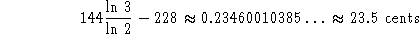This difference is so small that most people cannot hear it. (There are stories of violinists being particularly sensitive to such differences, however.)

Following up on the algebra of the preceding problem, we see that an interval corresponding to the ratio I equals 1200 log(I) cents. This will simplify the formulas given below for the other commas

There are other commas: The syntonic (or Didymic) comma is the difference between four perfect fifths and two octaves plus a major third. The syntonic comma occurs more easily than the Pythagorean comma or the schisma, since one doesn't need to go through particularly many chord progressions to move through four perfect fifths. The schisma is the difference between eight perfect fifths plus one major third and five octaves. The diaschisma is the difference between four perfect fifths plus two major thirds and three octaves . The computations are given below.

It should be clear at this point that most (indeed almost all) of the acoustic intervals will be imperfect in an equally tempered scale.

Let me come back to some of the details of even temperament after addressing another issue. Namely, why should we have twelve half steps in an octave anyway?

#### Continued Fractions

For convenience, denote the logarithm base 2 of x by log(x). The heart of our problem with fifths and octaves is the attempt to solve the equation 2^x = 3^y, where x and y are integers or rational numbers. Notice, if we're using rational numbers it is an equivalent problem to solve the equation 2^x = 3.

If I take logarithms base 2 of both sides of the troublesome equation, I am left with the equation

x log(2) = y log(3)

Of course, since log(2) = 1, the equation reduces to:

x = y log(3)

We then try to solve this for integer or rational values of x and y. Unfortunately, log(3) is not a rational number. The best we can do is to try to approximate it by a rational number. A decimal approximation is: 1.584962500721156181.

A good (and well-known) way to approximate an irrational number by a rational number is by continued fractions.

A continued fraction is an expression of the form: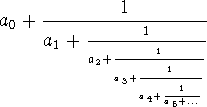whereare integers. Using this form (with only 1s in the numerators) means we will only be considering simple continued fractions.

For notational convenience, write [a_0, a_1, a_2, ...] for the infinite continued fraction above. Of course, it is also possible to consider finite continued fractions. It is an exercise to see that any rational number can be expressed as a finite continued fraction. I refer you to Hardy and Wright's book for a discussion of the uniqueness of such an expression. If we cut off an infinite continued fraction after N terms, we have the Nth convergent. For the infinite continued fraction given above, this iswhich is denoted. This is obviously a rational number, which we write (in reduced form) as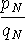There is a convenient algorithm for computing the continued fraction expansion of a given number x, called the continued fraction algorithm. For any positive number A, let [A] denote the integer part of A. To compute a continued fraction expansion for x, take a_0 = [x]. So

x = a_0 + x_0

and 0 <= x_0 < 1.

Now write

1/x_0 = a_1 + x_1   with  a_1 = [1/x_0]
and

1/x_1 = a_2 + x_2   with  0 <= x_2 < 1

and so on.

#### Some examples

In what follows, the notation for a repeating continued fraction is similar to that for a repeating decimal expression. For the continued fraction, we write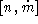.
•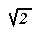=[1,2,2,2,...] =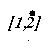with convergents:•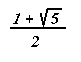=[1,1,1,1,1,...] =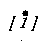with convergents: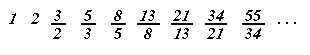Notice that the numerators and denominators of the convergents are successive Fibonacci numbers. If you start simplifying the convergents of the continued fraction [1,1,1,1,...] as a rational number, you will soon see why this is so. Also, it is a well-known fact that the ratio of successive Fibonacci numbers is indeed the golden mean. That is to say, this particular continued fraction does indeed converge to the irrational number it is supposed to represent.
• e = [2, 1, 2, 1, 1, 4, 1, 1, 6, 1, 1, 8, 1, 1, 10,...] with convergents:.
Remarkably, the obvious pattern in the continued fraction expansion for e actually persists.
•=[3,7,15,1,292,1,1,1,2,...] with convergents: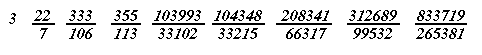.
Unlike the continued fraction expansion for e, the complete expansion foris unknown.

There are two pertinent theorems (see Hardy and Wright):

Theorem 1   If x is an irrational number and n >=1, thenSince the denominator of the (n+1)st convergent is strictly larger than the denominator of the nth convergent (and they are all integers), we see that the continued fraction expansion does indeed converge to the irrational number it is meant to be approximating.

Theorem 2   If x is an irrational number, n>=1, 0 < q <= q_n, and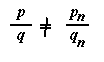with p, q integers, thenThat is to say, the nth convergent is the fraction among all fractions whose denominator is no greater than q_n which provides the best approximation to x. It is common to use the size of the denominator as a measure of the `complexity' of the rational number. Thus, we have that the nth convergent is optimal for a given complexity.

What does this say for our musical problem?

Recall that the troublesome equation 2^x = 3^y is equivalent to the equation 2^x = 3, provided we use rationals, and not just integers. The obvious solution is x=log(3). We want to approximate this by a rational number.

The continued fraction expansion for log(3) is [1,1,1,2,2,3,1,5,2,23,2,2,1,...] (see sequence A028507 of the On-Line Encyclopedia of Integer Sequences for more terms). The first few convergents are:

 1, 2, 3/2, 8/5, 19/12, 65/41, 84/53, 485/306
( A005663 / A005664).

Thus, taking the fourth approximation (start counting at zero):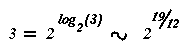That is to say, we obtain the perfect fifth, one octave up, by nineteen semitones. Moreover, the denominator, being twelve, forces us to have twelve semitones per octave. Thus, western music has adopted, quite by accident I assume, the fourth best approximation to a Pythagorean scale using equal temperament.

Obviously it is possible to have scales that come from dividing an octave into other than twelve pieces. For instance, the typical Chinese scale has five `notes' to the octave. Remarkably, this corresponds to the third convergent of the continued fraction expansion.

Going in the other direction, we could use the next more accurate continued fraction approximation of log(3), which would lead to an octave consisting of forty-one pieces. Below is a comparison of what happens to some standard intervals in these three systems. The fundamental interval for our standard twelve-tone chromatic scale is the semi-tone. There is no name for the basic intervals of our other chromatic scales. So we will refer to their basic intervals merely as `basic intervals'.

If we compute exactly in a twelve-tone scale, we find:

• The fifth isbasic intervals (semitones).
• The major third isbasic intervals (semitones).
• The minor third is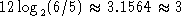basic intervals (semitones).

If we used a five-tone scale, we would have:

• The fifth being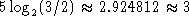basic intervals.
• The major third being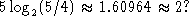basic intervals.
• The fourth beingbasic intervals.
• The minor third beingbasic intervals.
Thus, the major third and the perfect fourth would be indistinguishable in an equal-tempered five-tone scale.
The ? for the major third and minor third indicate that the rounding to the nearest integer is fairly inaccurate.

If we used forty-one semitones per octave, we would have:

• The fifth being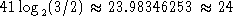basic intervals.
• The major third beingbasic intervals.
• The fourth beingbasic intervals.
• The minor third being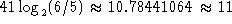basic intervals.
This type of scale has a fairly good separation of the standard acoustically distinct notes. I would guess that if we used such a scale, our ears would be trained to hear the difference between adjacent basic intervals. However, this difference is only 12/41, which is 29 cents, only slightly more than the Pythagorean comma.

Interestingly, around 40 B.C., King Fang, in China, discovered the sixth best approximation given above. It is unlikely, of course, that he actually used continued fractions to do this, which makes it all the more remarkable. In particular, Fang noticed that fifty-three perfect fifths are very nearly equal to thirty-one octaves. This leads to what is sometimes called the Cycle of 53. It can be represented by a spiral of fifths, replacing the more usual circle of fifths.### The Pythagorean Hammers and Acoustics

Western music has adopted certain intervals as basic to acoustics. It is not always the case that there the choices made are more natural than their alternatives. The legend about the source of some of these intervals involves Pythagoras. The story has him listening to the sound of the hammers of four smiths, which he found to be quite pleasant. Upon investigation, the hammers weighed 12, 9, 8, and 6 pounds. From these weights, Pythagoras derived the intervals:
• The octave:    12:6 = 2:1
• The perfect fifth:    12:8 = 9:6 = 3:2
• The perfect fourth:    12:9 = 8:6 = 4:3
• The whole step:    9:8

I don't know. Maybe. It's hard to say what really happened twenty-six centuries ago. But this certainly seems lucky. Maybe he was sitting in the same bath tub that Archimedes was sitting in four hundred years later.

In the present, we can look to see what might be natural intervals to construct. Firstly, the octave is quite natural, as a doubling of frequency. As usual, we will also take its inverse, halving of frequency, as equally natural. The next integral multiplication of frequency is tripling, which leads to the perfect fifth when combined with halving. Multiplying the frequency by four is just going up two octaves, so we already have that in our system.

The next natural operation is, then, to multiply the frequency by five. To remain in the original octave, we need to combine this with two halvings, leading to the interval of the major third.

Now it is not simply a preference for integers that leads to these intervals. There is also the phenomenon of overtones. A vibrating string has a fundamental tone, whose frequency f can be calculated from its length L, mass m and tension T according to a basic formula of acoustics:But the string also vibrates in other modes with less intensity. These other modes are vibrations at integer multiples of the fundamental frequency. The increasing sequence of such frequencies is called the harmonic series based on the given fundamental. The fundamental is called the first harmonic. The frequency of the octave (twice that of the fundamental) is called the second harmonic. The third harmonic is the perfect fifth one octave up from the fundamental. And so it goes. Thus, the argument for preferring intervals based on doubling, tripling and multiplying by five is actually based on acoustics, not just a fondness for the numbers 2, 3 and 5.

The phenomenon of overtones is an important factor in the quality of the sound of any particular instrument. Now, in theory, it may appear that the harmonic series for a particular fundamental frequency continues through all the integers. However, this would surely produce unbearable dissonance. What actually happens is that the intensity of the higher harmonics decreases quite rapidly. Indeed, on some instruments it is difficult to discern beyond the third harmonic. (My guitar, for instance.) Violins and oboes have strong higher harmonics, leading to a `bright' tone. Flutes and recorders have weak higher harmonics. Apparently the clarinet has strong odd-numbered harmonics, which is why has a `hollow' tone. Before valves were added to brass instruments, it was only notes corresponding to harmonics that could be played on these instruments.

After the intervals based on multiplying by two, three and five, our choices become more arbitrary.

• The perfect fourth. Should we go down a perfect fifth then up an octave, resulting in an interval of (3/2)(2)=4/3? Or should we do something else?
• The whole tone. Why is it better to go up two perfect fifths and down an octave: (3/2)(3/2)(1/2)=9/8 rather than, say, up two fifths and down three major thirds: (3/2)(3/2)*(4/5)(4/5)(4/5) = 144/125? (There is a difference of about 41 cents here.)
• The minor third. Should we use (4/5)(3/2) = 6/5, i.e. down a major third and up a perfect fifth, or (2/3)(2/3)(2/3)(2)(2) = 32/27, i.e. down three fifths and up two octaves?
• The major third. One could even argue that (3/2)(3/2)(3/2)(3/2)(1/2)(1/2) = 81/64 is preferable to 5/4 as the former is obtained by going up four perfect fifths then down two octaves, thus using only the doubling and tripling rules.For the sake of curiosity, we could investigate what we obtain using the major third as the basis for our computations. The acoustic major third is 5/4. Thus, the critical quantity is log(5/4) = log(5) - log(4).

Since log(4) is an integer, the crux of the approximation is that of log(5). The continued fraction expansion is [2, 3, 9, 2, 2, 4, 6, 2, 1, 1, 3, 1, 18] The convergents are: 7/3, 65/28, 137/59, 339/146, 1493/643, ...

Since three is certainly too few for an octave, we would have been stuck with octaves of twenty-eight notes!#### Some other commas

Syntonic Comma
The syntonic (or Didymic) comma is the difference between four perfect fifths and two octaves plus a major third.

Four perfect fifths correspond to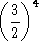. In the key of C, this is.

Two octaves plus a major third correspond to.

Using the logarithmic scale,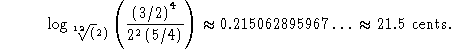Schisma
The schisma is the difference between eight perfect fifths plus one major third and five octaves.

Eight perfect fifths plus one major third correspond to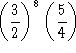.

Five octaves correspond to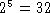.

Using the logarithmic scale: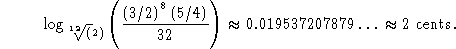Diaschisma
The diaschisma is the difference between four perfect fifths plus two major thirds and three octaves . The computation is#### Mean-tone system

One alternative to equal temperament is the mean-tone system, which seems to have begun around 1500. In mean temperament, the fifth is 697 cents, as opposed to 700 cents in equal temperament or 701.955 cents for the acoustically correct interval. The mean-tone system for tuning a piano is satisfactory in keys that have only one or two sharps or flats. But there are problems. For instance, G#=772 cents and Ab=814. They ought to be the same! This discrepancy is called the wolf. While the Pythagorean comma, at 23.5 cents, is not discernible by most listeners, the wolf, at 52 cents is quite noticeable.

Before equal temperament was widely accepted, keyboards had to accommodate these problems. One solution was only to play simple pieces in the keys your instrument could handle. A second solution, which was certainly necessary for large and important organs, was to have divided keyboards. Thus, the single key normally used today for G# and Ab would be split into two keys. Often, the back of one key would be slightly raised to improve the organist's ability to play by touch. The most extraordinary keyboard I was able to find a reference to was Bosanquet's `Generalized Keyboard Harmonium' built in 1876, which had 53 keys per octave!

#### Acknowledgment

My thanks to Mark McConnell for his help with the ideas in this web page.

#### References

• Eric Blom (ed.) Grove's Dictionary of Music and Musicians, Fifth Edition, St Martin's Press, Inc., 1955.
• G.H. Hardy and E.M. Wright, An Introduction to the Theory of Numbers, Fourth Edition, Oxford University Press, 1975.
See, in particular, Chapters X and XI concerning continued fractions.
• Don Michael Randel, Harvard Concise Dictionary of Music, Harvard University Press, 1978.
See the sections titled `Comma' and `Temperament'.
• Percy Scholes, The Oxford Companion to Music, Ninth Edition,Oxford University Press, 1955.
See the section titled `Temperament'.

#### Definitions

Chromatic Scale
The chromatic scale contains all the possible pitches in an octave, as opposed to a diatonic scale, which contains combinations of whole tones and semitones. When using octaves divided into other than twelve intervals, the chromatic scale contains all the microtones in the subdivision.
Semitone
A semitone is the basic interval of the standard octave of western music. That is to say, it is an interval of 2^(1/12). For the scales of five, twelve and forty-one notes that are also considered here, the semitone is not quite as useful. Instead, we speak of the `basic interval'. For the scale obtained by dividing the octave into five pieces, the basic interval is 2^(1/5). Generally, intervals that are not obtained from semitones are called microtones.
Tonic
The tonic is the first note in a key or scale. It is also the note after which the scale is named, hence, the keynote.
Major Third
For the purposes of this discussion, we take a major third to be defined as the interval corresponding to a change in frequency by a factor of 5/4. In the key of C, this is the interval C-E (but only approximately in equal temperament!).
Minor Third
For the purposes of this discussion, we take a minor third to be defined as the interval corresponding to a change in frequency by a factor of 6/5. In the key of C, this is the interval (approximated by!) C-Eb.
Temperament
For our purposes, temperament refers to any system of defining the frequencies of the notes in a scale, be it chromatic, diatonic or some other sort of scale.

Edward Dunne (egd@ams.org)
American Mathematical Society